# RS Aggarwal Solutions for Class 6 Chapter 7 Decimals Exercise 7B

RS Aggarwal Solutions for Class 6 Chapter 7 Decimals Exercise 7B consists of questions related to converting decimals into fractions and vice versa along with the general methods followed in solving them. Mathematics plays an important role in students’ life as it is one of the scoring subjects. RS Aggarwal Solutions improves the problem solving abilities among the students. These solutions are so perfect that students who practice them will clear their doubts with ease. Practising RS Aggarwal Solutions improves students’ performance in the examinations. PDF can be downloaded from the links which are available here.

## Download PDF of RS Aggarwal Solutions for Class 6 Chapter 7 Decimals Exercise 7B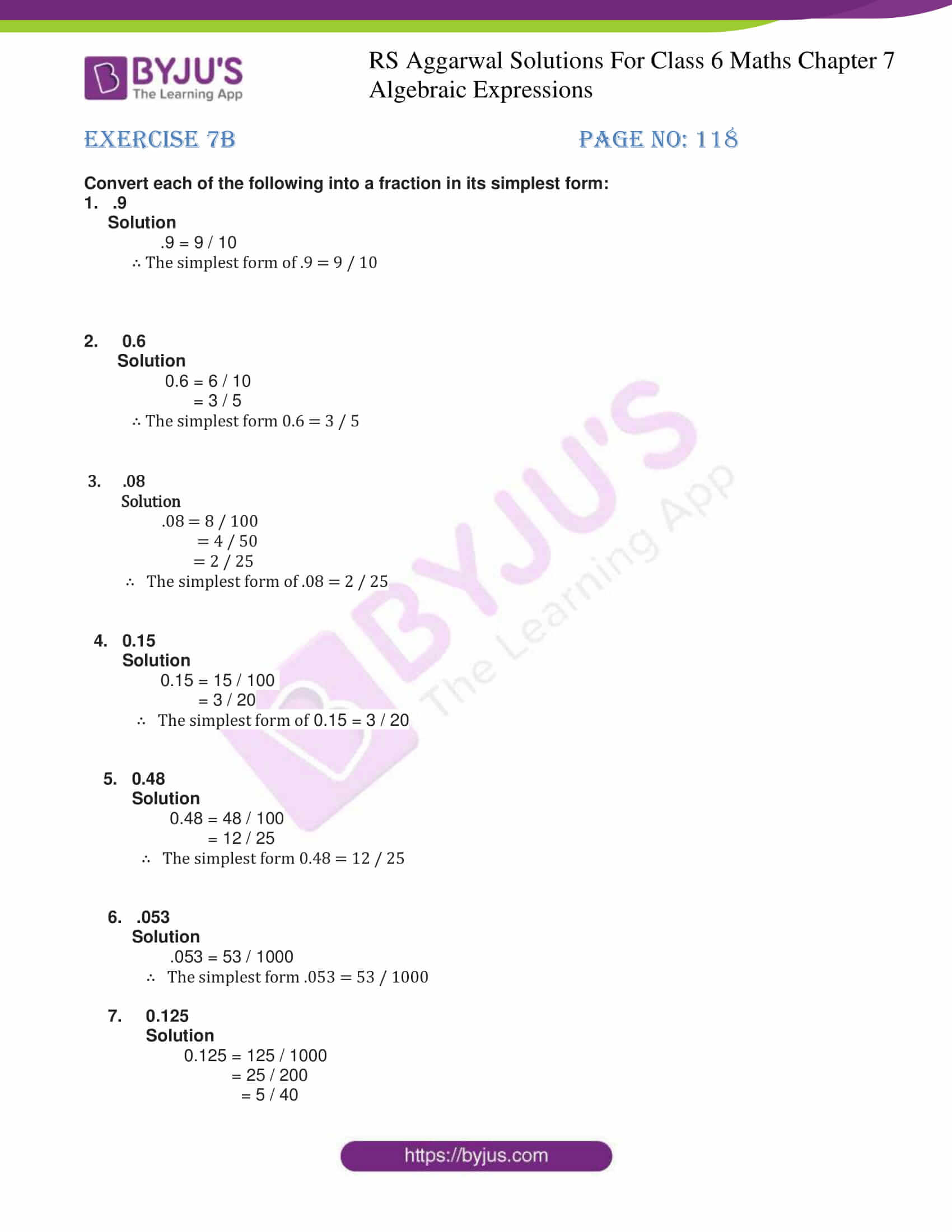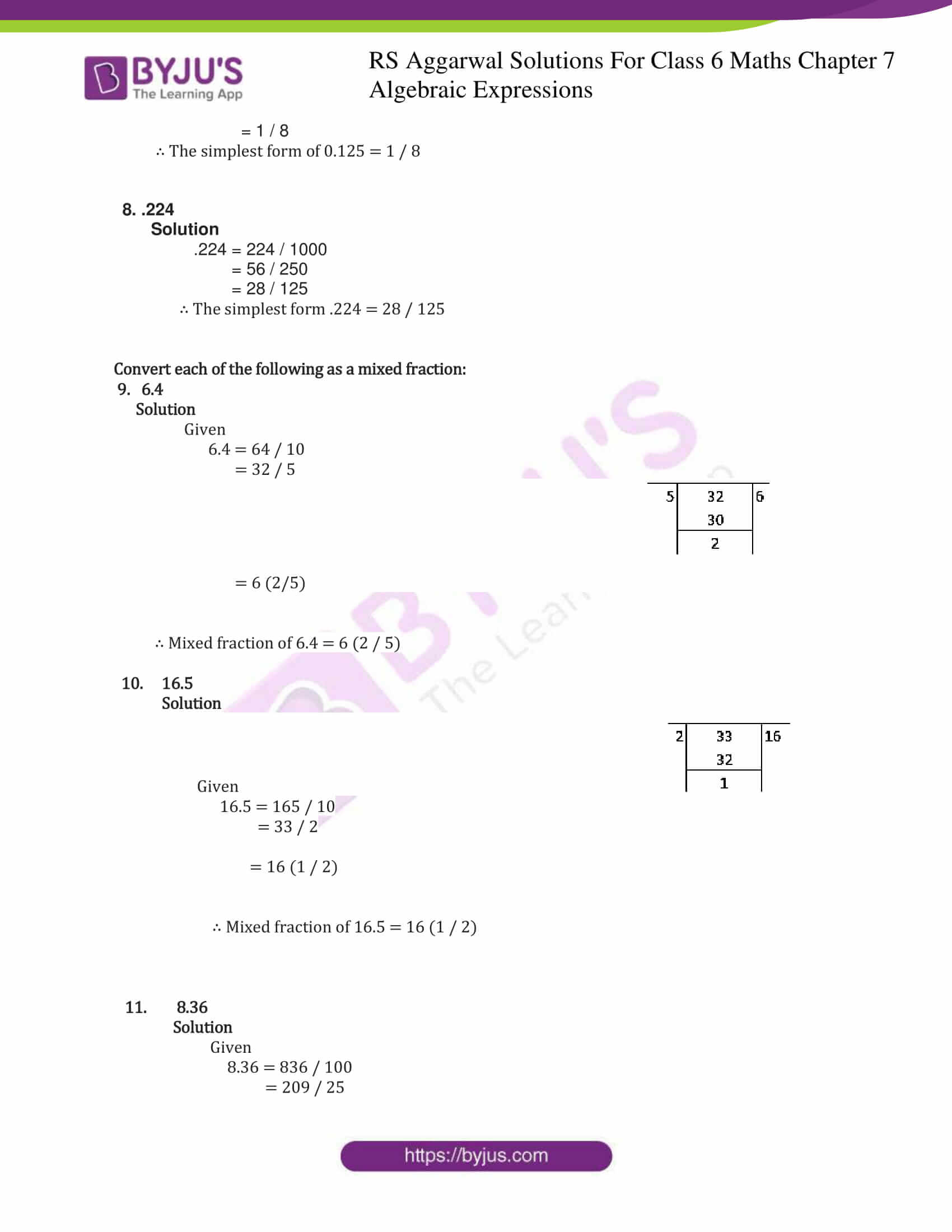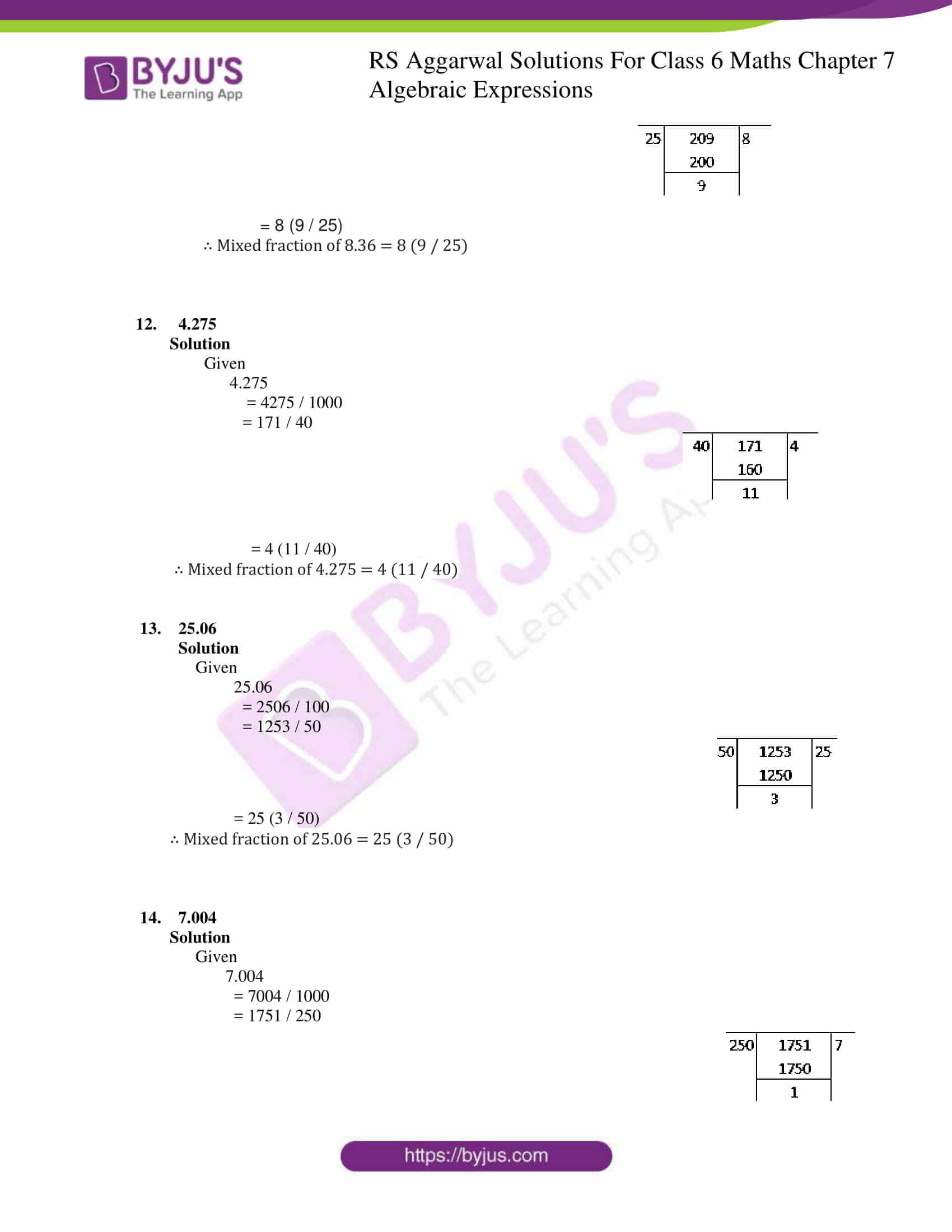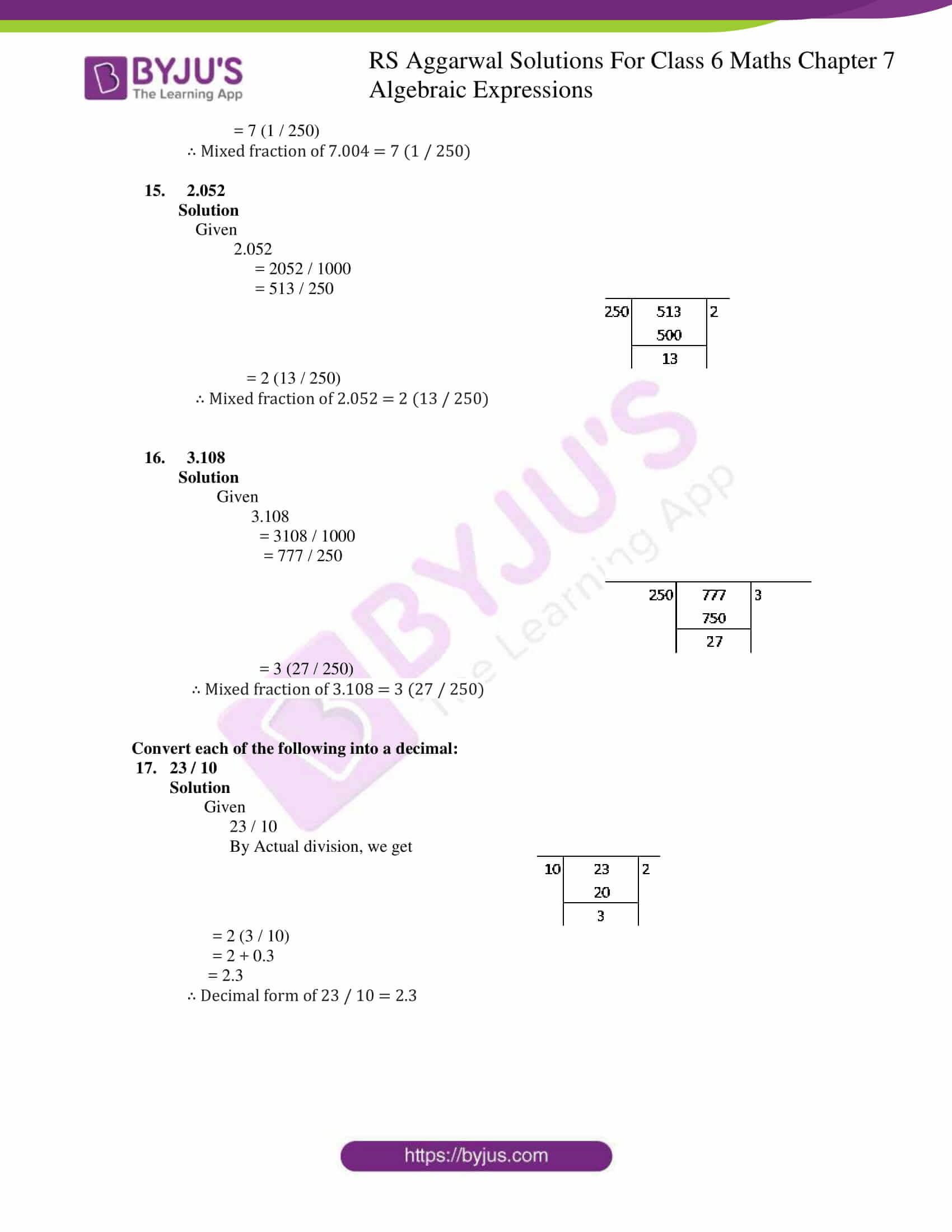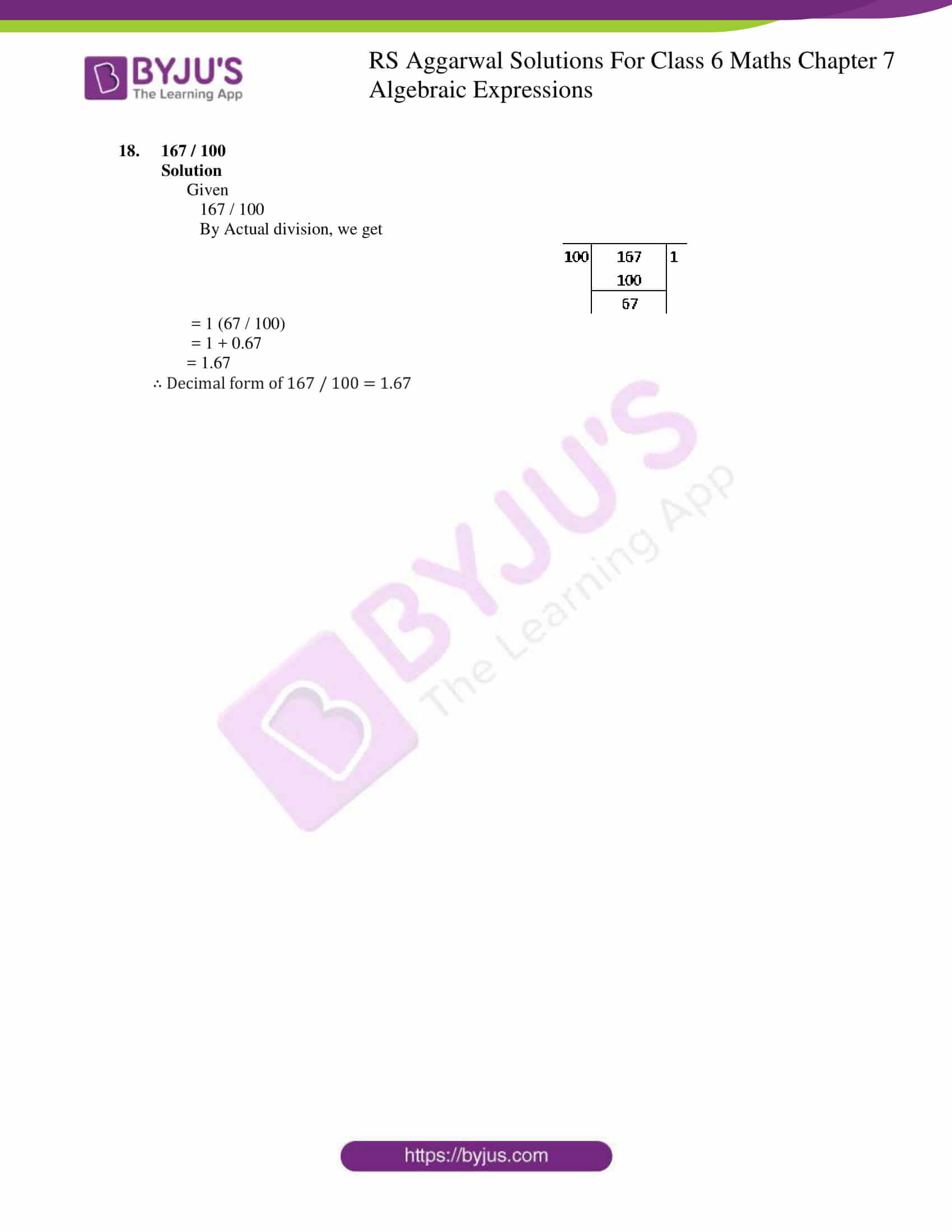### Access answers to Maths RS Aggarwal Solutions for Class 6 Chapter 7 Decimals Exercise 7B

Convert each of the following into a fraction in its simplest form:

1. .9

Solution

.9 = 9 / 10

∴ The simplest form of .9 = 9 / 10

2. 0.6

Solution

0.6 = 6 / 10

= 3 / 5

∴ The simplest form 0.6 = 3 / 5

3. .08

Solution

.08 = 8 / 100

= 4 / 50

= 2 / 25

∴ The simplest form of .08 = 2 / 25

4. 0.15

Solution

0.15 = 15 / 100

= 3 / 20

∴ The simplest form of 0.15 = 3 / 20

5. 0.48

Solution

0.48 = 48 / 100

= 12 / 25

∴ The simplest form 0.48 = 12 / 25

6. .053

Solution

.053 = 53 / 1000

∴ The simplest form .053 = 53 / 1000

7. 0.125

Solution

0.125 = 125 / 1000

= 25 / 200

= 5 / 40

= 1 / 8

∴ The simplest form of 0.125 = 1 / 8

8. .224

Solution

.224 = 224 / 1000

= 56 / 250

= 28 / 125

∴ The simplest form .224 = 28 / 125

Convert each of the following as a mixed fraction:

9. 6.4

Solution

Given

6.4 = 64 / 10

= 32 / 5

= 6 (2/5)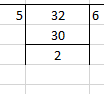∴ Mixed fraction of 6.4 = 6 (2 / 5)

10. 16.5

Solution

Given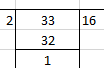16.5 = 165 / 10

= 33 / 2

= 16 (1 / 2)

∴ Mixed fraction of 16.5 = 16 (1 / 2)

11. 8.36

Solution

Given

8.36 = 836 / 100

= 209 / 25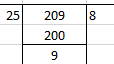= 8 (9 / 25)

∴ Mixed fraction of 8.36 = 8 (9 / 25)

12. 4.275

Solution

Given

4.275

= 4275 / 1000

= 171 / 40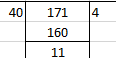= 4 (11 / 40)

∴ Mixed fraction of 4.275 = 4 (11 / 40)

13. 25.06

Solution

Given

25.06

= 2506 / 100

= 1253 / 50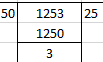= 25 (3 / 50)

∴ Mixed fraction of 25.06 = 25 (3 / 50)

14. 7.004

Solution

Given

7.004

= 7004 / 1000

= 1751 / 250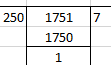= 7 (1 / 250)

∴ Mixed fraction of 7.004 = 7 (1 / 250)

15. 2.052

Solution

Given

2.052

= 2052 / 1000

= 513 / 250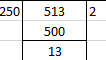= 2 (13 / 250)

∴ Mixed fraction of 2.052 = 2 (13 / 250)

16. 3.108

Solution

Given

3.108

= 3108 / 1000

= 777 / 250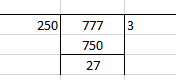= 3 (27 / 250)

∴ Mixed fraction of 3.108 = 3 (27 / 250)

Convert each of the following into a decimal:

17. 23 / 10

Solution

Given

23 / 10

By Actual division, we get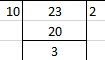= 2 (3 / 10)

= 2 + 0.3

= 2.3

∴ Decimal form of 23 / 10 = 2.3

18. 167 / 100

Solution

Given

167 / 100

By Actual division, we get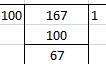= 1 (67 / 100)

= 1 + 0.67

= 1.67

∴ Decimal form of 167 / 100 = 1.67